# Buck Converter Duty Cycle Derivation

In this article I will share to you the methods I used in buck converter duty cycle derivation. We will consider the ideal and the real scenarios. In ideal buck converter duty cycle derivation, you don’t need to consider the voltage drops of the switching element and catch diode. On the other hand, in real buck converter duty cycle derivation, the voltage drops are taken into account.

Below is a typical buck converter circuit diagram. In a conventional buck converter the PWM is applied to the switching device which is an N-channel MOSFET in the figure below.

`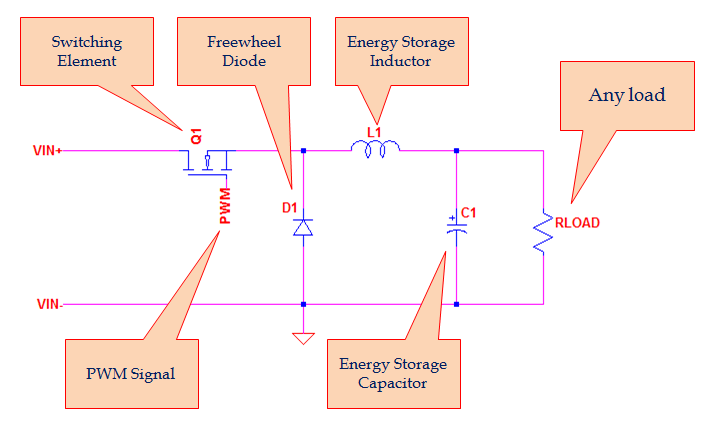`

Below are the current waveforms of a buck converter. These include the MOSFET current in green, diode current in yellow and the inductor current in red. The bottom waveform in blue is the PWM signal. I will discuss here two ways of formulating the duty cycle of a buck converter. The first one is to use the inductor voltage/current equation then do integration. The second one is to use volt-second balance method. Actually buck converter duty cycle and currents are all can be derived from its waveform. Thus it is very important to know the waveforms. Below are the waveforms of buck converter currents operating in CCM.

## Buck Converter Duty Cycle Derivation using the Inductor Current Waveform

Now, let’s start to derive the buck converter duty cycle using the inductor current waveform above.

This is very important. This buck converter duty cycle derivation is only valid for operation in CCM and boundary mode. For DCM, this must not be used.

``

When the PWM signal is high, the inductor current will ramp up linearly as above waveform. When the PWM signal is low, the inductor current will decay in a linear fashion. So, the level of the current rise and current decay is the same.

#### Analysis at Ton

The current rise is can be expressed as

`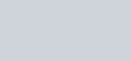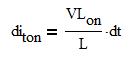`

Integrate to eliminate dt

``
``

For a pulse or a PWM signal, the time the logic is high (Ton) divided by the period (Tsw) is called the duty cycle.

`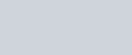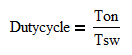`

Expressing Ton

`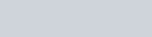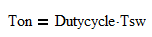`

Substitute Ton to the diton equation

`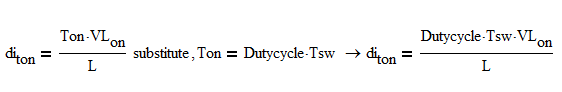`
`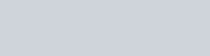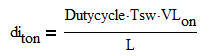`

During PWM high, the MOSFET Q1 will turn on and the voltage loop will be

`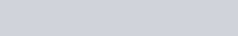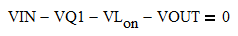`

Expressing VLon

`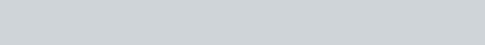`

Substitute to diton will give

`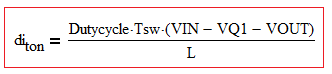`

#### Analysis at Toff

The decay current is expressed by the equation

`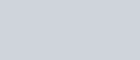`

Integrate to eliminate dt

``
``

Replace Ton by

``

Then

`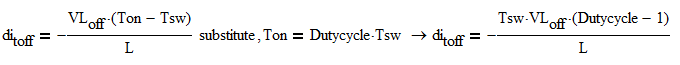`
``

During PWM low, the MOSFET turns off and the inductor will reverses its polarity and the diode will be forward biased. The voltage loop will be

`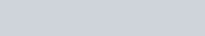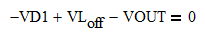`

Expressing VLoff

`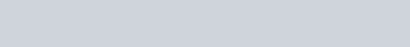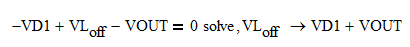`

Substitute to ditoff will give

`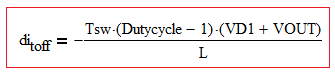`

Equating diton and ditoff

The final stage of this buck converter duty cycle derivation is to equate the inductor rise and decay currents derived above.

`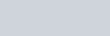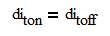`
``
`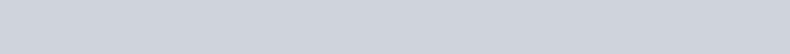`

And the real buck converter duty cycle is

``

Considering the voltage drop of the MOSFET and diode are zero,

the ideal duty cycle will be

``

Where;

VD1 – voltage drop of the diode

VOUT – buck converter output voltage

VQ1 – MOSFET voltage drop

VIN – input voltage of the buck converter

## Buck Converter Duty Cycle Derivation using Volt-Second Balance Concept

This approach is actually a short cut to the previous method. This concept makes use of the product of inductor voltage and time. The product of the inductor voltage during PWM high and Ton is equal to the product of the inductor voltage during PWM low or Toff as below figure shows.Waveform used in buck converter duty cycle derivation using the volt-second balance method

Mathematically,

`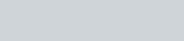`

The inductor voltages during Ton and Toff are given below as derived in the first method.

`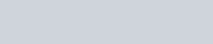`
`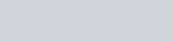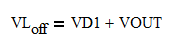`

So

`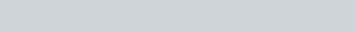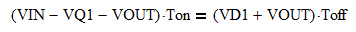`

Toff is can be expressed as

`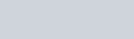`

Substitute to above equation will give

`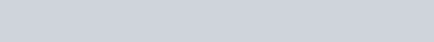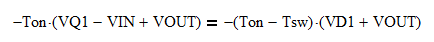`

Substitute Ton

`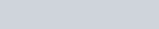`

The result will be

`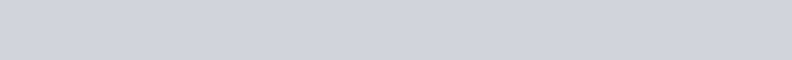`

The duty cycle equation is therefore

``

Considering ideal values

``

The result is very the same to the previous method.

## Conclusion

Buck converter duty cycle derivation is can be done through the inductor current waveform and then perform integration. This is the long method. To get the duty cycle, the inductor current linear ramp and decay will be equated.

This technique is very fundamental and requires great analytical skills. However, once you master this out, you can derive duty cycles of any topology. Another technique that can be used for Buck converter duty cycle derivation is using volt-second method. Actually this is a short cut on the fundamental approach and somehow derived from it. Whichever method you will use will give you the same result.